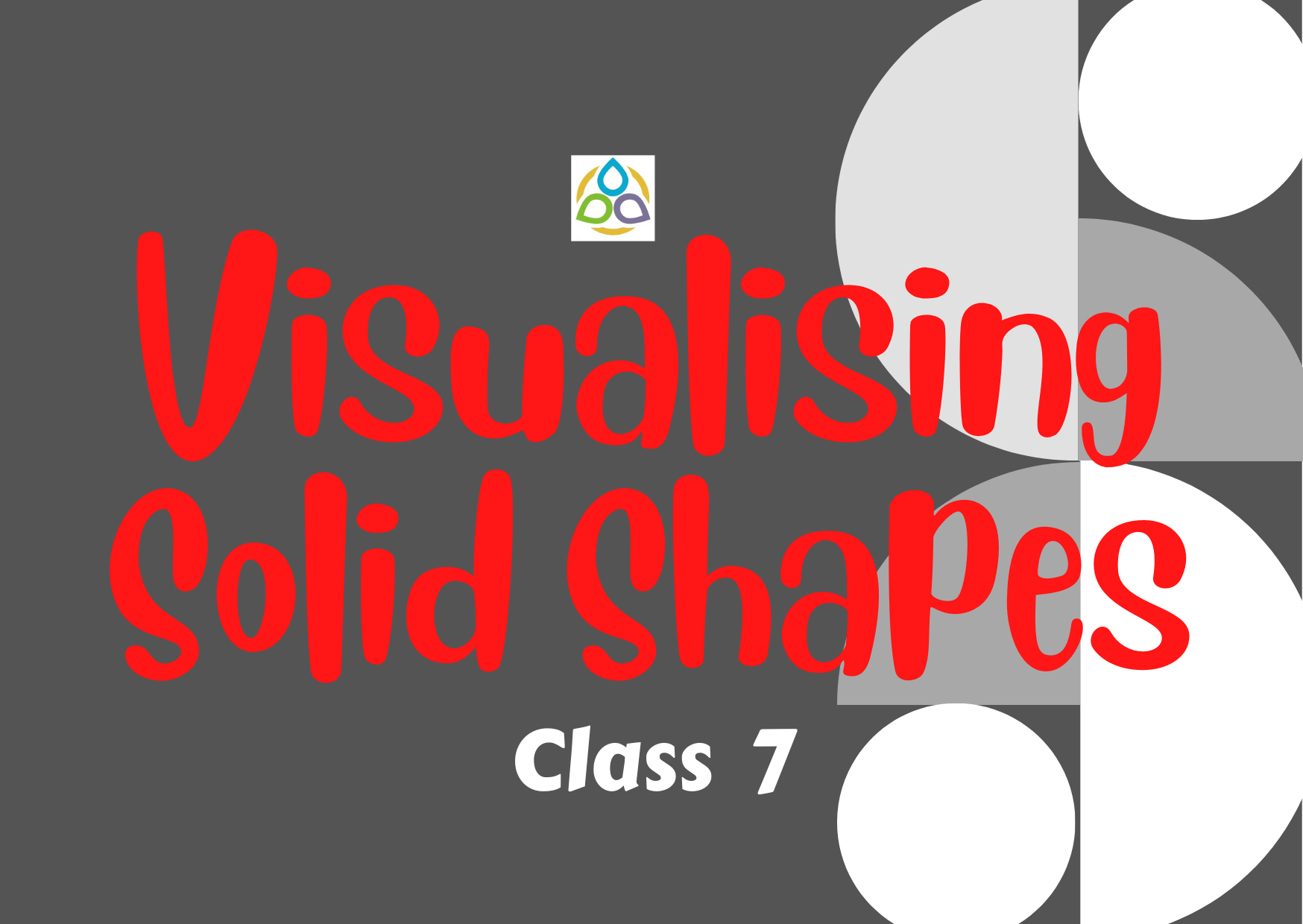# Visualising Solid Shapes – 7

Multiple Choice Questions

75. Plane figures are of

• (A) Two dimensions
• (B) Three dimensions
• (C) One dimension
• (D) Four dimensions

74. Solid shapes are of

• (A) Two dimensions
• (B) Three dimensions
• (C) One dimension
• (D) Four dimensions

73. The corners of a solid shape are called its

• (A) Faces
• (B) Edges
• (C) Vertices
• (D) Flat surface

72. The line segments/ curves which form the skeleton of the solid shapes are known as its

• (A) Faces
• (B) Edges
• (C) Vertices
• (D) Flat surface

71. The flat surfaces of a solid shape are called its

• (A) Faces
• (B) Edges
• (C) Vertices
• (D) Flat surface

70. Which of the following is a two Dimensional figure?

• (A) Rectangle
• (B) Rectangular Prism
• (C) Square Pyramid
• (D) Square Prism

69. Which of the following can be the base of a pyramid?

• (A) Line segment
• (B) Circle
• (C) Octagon
• (D) Oval

68. The skeleton outline of a solid that can be folded to make the solid is known as

• (A) Faces
• (B) Edges
• (C) Vertices
• (D) Net

67. How many types of sketches of a solid are possible?

• (A) 0
• (B) 1
• (C) 2
• (D) 3

66. The sketch of a solid shape which does not have proportional measurements is known as

• (A) Oblique sketch
• (B) Isometric sketch
• (C) Slicing

65. The sketch of a solid shape in which the measurements are kept proportional is known as

• (A) Oblique sketch
• (B) Isometric sketch
• (C) Slicing

64. An isometric dot paper is used to draw

• (A) An oblique sketch
• (B) An isometric sketch
• (C) Slicing

63. How many vertices are there in a cube?

• (A) 8
• (B) 12
• (C) 6
• (D) 10

62. How many edges are there in a cube?

• (A) 8
• (B) 12
• (C) 6
• (D) 10

61. How many faces are there in a cube?

• (A) 8
• (B) 12
• (C) 6
• (D) 10